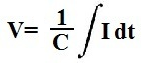﻿ Capacitor Voltage Calculator ﻿# Capacitor Voltage CalculatorExample Currents To Enter
5sin(60t)
10cos(110t)
15sin(120t)

This Capacitor Voltage Calculator calculates the voltage across a capacitor based on the current, I, flowing through the capacitor and the capacitance, C, of the capacitor.

The formula which calculates the capacitor voltage based on these input parameters is V= 1/C∫Idt, where V is equal to the voltage across the capacitor, C is equal to the capacitance of the capacitor, and I is equal to the current flowing through the capacitor. Many times, you will see the extended formula, V= V0 + 1/C∫Idt. This formula takes into account any initial voltage that may be across the capacitor to begin with. However, for the sake of simplicity, we have made this calculator not accept initial conditions. If you have initial conditions, just simply add that value with the value that this calculator computes.

The integral of the current, I, really means that the sum of all the currents are added up with respect to time.

The current can be a DC or AC value. If it is DC, a numerical value is entered. If it is AC, the value will be a sine or a cosine waveform. A sine or cosine waveform replicates an AC signal which may be seen in real life such as the AC power which comes out of a wall outlet. So values such as sin(60t), 10sin(120t), 15cos(110t) can be entered into the calculator. And the resultant integral will be computed for it.

To use this calculator, a user simply enters the current, I, capacitance, C. The user can decide if s/he wants the answer computed in fractional form or decimal form. S/he then clicks the 'Calculate' button, and the resultant voltage value in unit volts is automatically computed and displayed.

Examples

What is the voltage across a capacitor if the voltage is 6sin(60t) and the capacitance is 2F?

V= 1/C∫Idt= (1/2F)∫(6sin(60t))= -0.05cos(60t) V

So the current flowing across the capacitor is -0.05cos(60t) V.

What is the voltage across a capacitor if the voltage is 5cos(60t) and the capacitance is 5F?

V= 1/C∫Idt= (1/5F)∫(5cos(60t))= 0.0166666666667sin(60t) V

So the current flowing across the capacitor is 0.0166666666667sin(60t)

Related Resources

﻿